Home > CC4 > Chapter 11 > Lesson 11.2.3 > Problem11-82

11-82.
1. Examine the triangle pairs below, which are not necessarily drawn to scale. For each pair, determine: Homework Help ✎

• If they must be congruent. If they are congruent, write a correct congruence statement (such as ΔPQR ≅ ΔSTU) and state the congruence property (such as SAS ≅).

• If there is not enough information or if they cannot be congruent, explain why you cannot ensure congruence.

2.  a.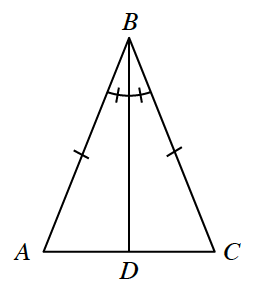b.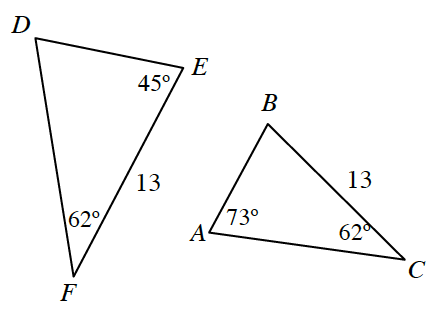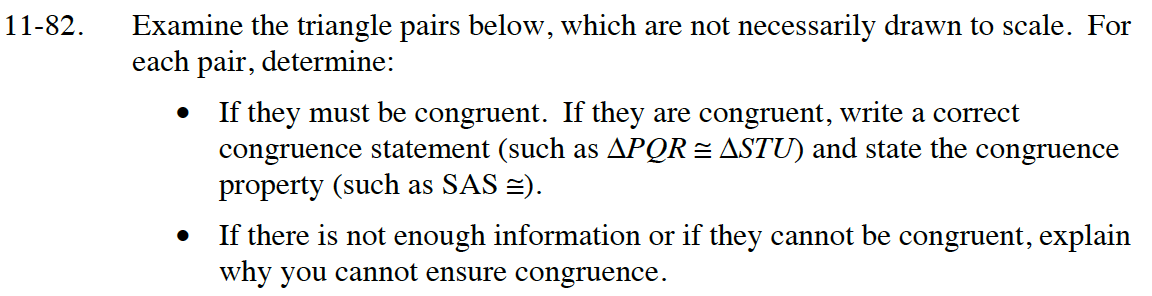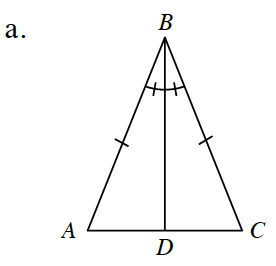Review the Math Notes box in section 7.1.7.
The shared side BD is congruent to itself by the Reflexive property.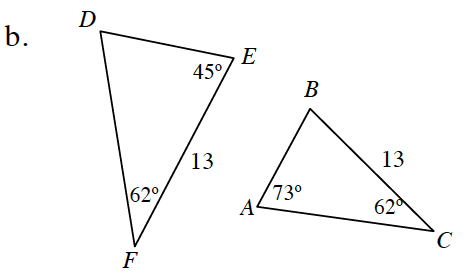Calculate the missing angle for each triangle.
Review the Math Notes box in section 7.1.7.